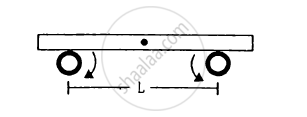Department of Pre-University Education, KarnatakaPUC Karnataka Science Class 11
Advertisement Remove all ads

# A Uniform Plate of Mass M Stays Horizontally and Symmetrically on Two Wheels Rotating in Opposite Direction in Figure . - Physics

Sum

A uniform plate of mass M stays horizontally and symmetrically on two wheels rotating in opposite direction in Figure . The separation between the wheels is L. The friction coefficient between each wheel and the plate is μ. Find the time period of oscillation of the plate if it is slightly displaced along its length and released.Advertisement Remove all ads

#### Solution

Let x be the displacement of the uniform plate towards left.
Therefore, the centre of gravity will also be displaced through displacement x.
At the displaced position,
R1 + R2 = mg
Taking moment about g, we get:

$R_1 \left( \frac{L}{2} - x \right) = R_2 \left( \frac{L}{2} + x \right) = \left( Mg - R_1 \right) \left( \frac{L}{2} + x \right) . . . . \left( 1 \right)$

$\therefore R_1 \left( \frac{L}{2} - x \right) = \left( Mg - R_1 \right) \left( \frac{L}{2} + x \right)$

$\Rightarrow R_1 \frac{L}{2} - R_1 x = Mg\frac{L}{2} - R_1 x + Mgx - R_1 \frac{L}{2}$

$\Rightarrow R_1 \frac{L}{2} + R_1 \frac{L}{2} = Mg\left( x + \frac{L}{2} \right)$

$\Rightarrow R_1 \left( \frac{L}{2} + \frac{L}{2} \right) = Mg\left( \frac{2x + L}{2} \right)$

$\Rightarrow R_1 L = Mg\left( 2x + L \right)$

$\Rightarrow R_1 = Mg\frac{\left( L + 2x \right)}{2L}$

$\text { Now },$

$F_1 = \mu R_1 = \mu Mg\frac{\left( L + 2x \right)}{2L}$

$\text { Similarly },$

$F_2 = \mu R_2 = \mu Mg\frac{\left( L - 2x \right)}{2L}$

$\text { As } F_1 > F_2 , \text { we can write: }$

$F_1 - F_2 = Ma = \left( 2\mu\frac{Mg}{L} \right)x$

$\frac{a}{x} = 2\mu\frac{g}{L} = \omega^2$

$\Rightarrow \omega = \sqrt{\frac{2\mu g}{L}}$

$\text { Time period }\left( T \right)\text{ is given by },$

$T = 2\pi\sqrt{\frac{L}{2\mu g}}$

Is there an error in this question or solution?
Advertisement Remove all ads

#### APPEARS IN

HC Verma Class 11, 12 Concepts of Physics 1
Chapter 12 Simple Harmonics Motion
Q 31 | Page 254
Advertisement Remove all ads

#### Video TutorialsVIEW ALL 

Advertisement Remove all ads
Share
Notifications

View all notifications

Forgot password?